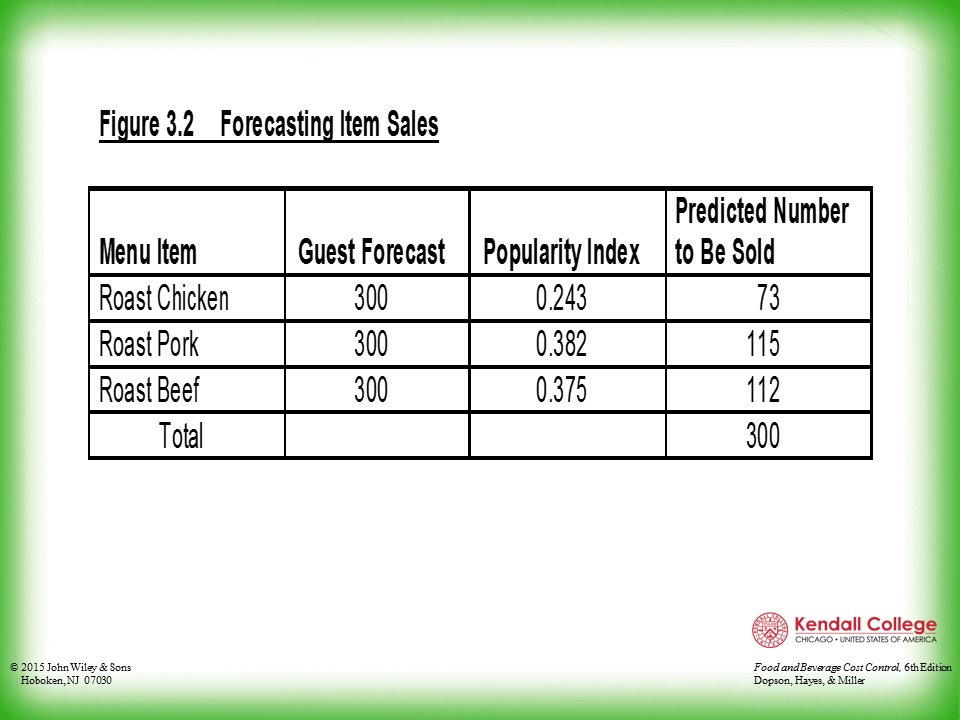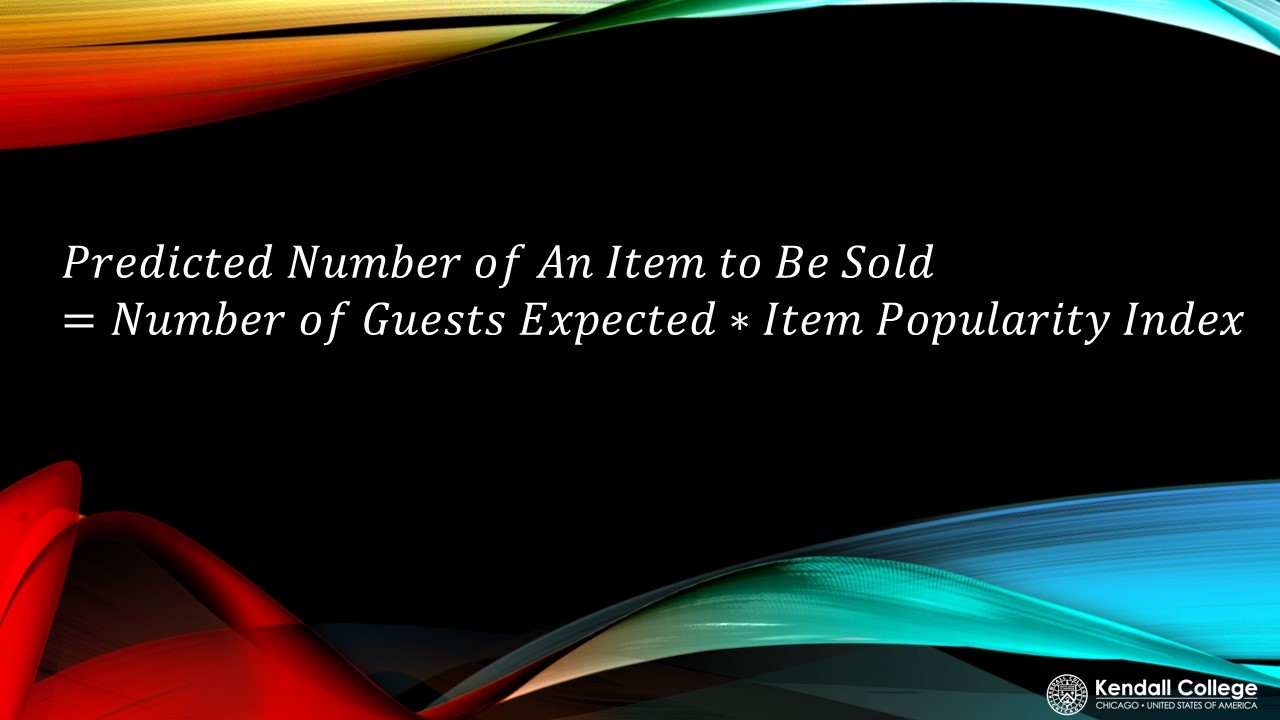# COST CONTROL: POPULARITY INDEX

This guide gives an overview of cost control and includes a link to a YouTube channel that is very helpful for saving money

## INTRODUCTION

As a manager, we want to minimize expenses and maximize profit. One way of doing that is by making sure that menu production is as close as possible to what customer’s order. By using data collected over time, we can apply the percentage of an item ordered during a meal period. This helps the manager decide what amounts are appropriate, so the restaurant does not run out of ingredients or have excessive amounts.

## WHAT IS A POPULARITY INDEX?

The word “popularity” can be used to remember the definition of this term. In other words, the popularity index is how “popular” is an item on the menu or the percentage of total guests choosing a given menu item from a list of alternatives.

Here’s a Similar Popularity Index:source

## EXAMPLE OF POPULARITY INDEX IN ACTION!

Using the formulas given below, see if you can figure out how they calculated the popularity index in Figure 3.2 and the Predicted Number to Be Sold.## STRATEGIES/TIPS TO REMEMBER

1. The most effective way to understand and become more comfortable with Cost Control is to complete the practice on paper AND on Excel.
2. Look for patterns and keywords with in terms that tell you how to calculate the given piece of information. For example, the word “per” means division. You want to divide the word in front of “per” and divide it by the word after the word “per.” Sales per Guest means sales divided by guest.
3. Lastly, understand the purpose of each data point. This can help you remember how to calculate the data or value. For example, revenue forecast represents a future amount or value, therefore, we used data that predicts the future like an increase estimate. Also, the word “forecast” in revenue forecast gives us a hint that it is telling what the future might be.

## ASK THE ASC FOR HELP!## TWO IMPORTANT FORMULAS

Popularity Index:Predicting Number of Item to Be Sold:## LET'S SEE POPULARITY INDEXING IN ACTION!

Examples by hand:

Examples in Excel: